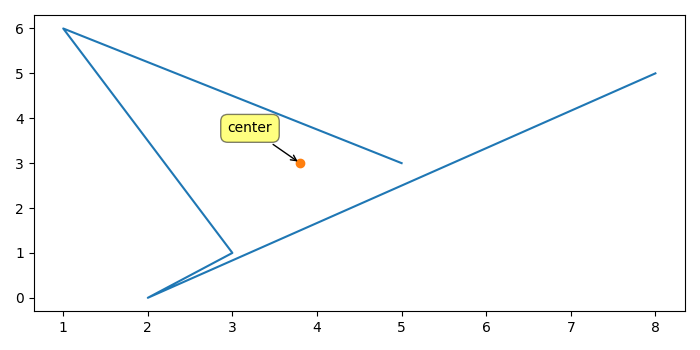# How to get the center of a set of points using Python?

MatplotlibPythonData Visualization

To get the center of a set of points, we can add all the elements of the list and divide that sum with the length of the list so that result could be the center of the corresponding axes.

## Steps

• Make two lists of data points.

• Plot x and y data points using plot() method.

• Get the center tuple of the x and y data points.

• Place the center point on the plot.

• Annotate the center as label for center of the x and y data points.

• To display the figure, use show() method.

## Example

from matplotlib import pyplot as plt
plt.rcParams["figure.figsize"] = [7.00, 3.50]
plt.rcParams["figure.autolayout"] = True
x = [5, 1, 3, 2, 8]
y = [3, 6, 1, 0, 5]
plt.plot(x, y)
center = sum(x)/len(x), sum(y)/len(y)
plt.plot(center, center, marker='o')
plt.annotate(
"center",
xy=center, xytext=(-20, 20),
textcoords='offset points', ha='right', va='bottom',
plt.show()